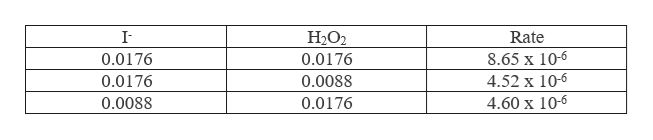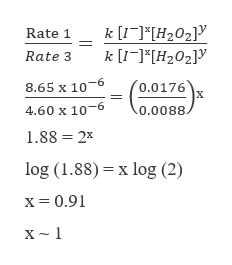o0TEXASS Phan#137144DistribAll contents of the reaction flasks may be disposed of into the sink.al:lations:Aew the method of initial rates in yur text. You will need the values for the initial concentrations of each reactant asell as the rate.Hypothetical data for reaction: A+2B C+ 2DRun[Alo[BloInitial A[C]/At (Molarity/sec)10.150 M0.150 M8.0020.150 M0.300 M16.030.300 MRate = k[A]* [B]. We need to calculate x, y and k0.150 M32.0To get x, we have to hold [B] constant and just see how [A] affects the rate. Take the ratio of Run 3/Run 1Run 3Initial ΔΙCI/ΔtInitial A[C]/At32.0 M/sec8.0 M/seck[Al[B]Yk[A]* [B]Yk(0.300M)x (0.150M)k(0.150M)* (0.150 M)XRun 1YSimplifying:4.0 (0.150M) * / (0.150M) *4.0 (0.300/0.150)x or 4.0 = 2* Or x 2orDo the same for B (holding [A] constant) and discover that y = 1.You can calculate k as well. Using run 1:8.00 M/sec k (0.150M)1 (0.150M)1and solving for k, we get k = 356 M1 s1'k' can be calculated using any of the three runs from Part IFor your data:Find x and y as explained above. Calculate k for each run and average the three values at the end. If your values for xand y do not turn out to be whole numbers, round to the nearest whole number. Write the Rate Law using your valuesfor x, y and k:= k [IF]* [H2O2]'Rate CVSOSAGCa20ou ud SreTEXAS INSTRUMENTSDistribjock Reaction Part 1: PostLabNameUsing your calcuations from your pre-lab, how many moles of S2O3 are consumed in the experiment?=cocoz5 mol s203300.OIOM S203=M9rYol sz OO023 LS4O62(aq). How many moles of 12 will react with the 2YI2. Given that: 2 (aq) + 2 S2O32(aq)21 (aq).25L2-S 1a s.2.S adOmoles of S2O32-?mol szoe?M:molesZmo1s203moiesMxLCalculate the molarity of l2 that reacted [All: (divide moles reacted by total reaction volume) (42.5mL)3./.0425) L(1.25x 10Smo)2.94x 10ALT2JFill in the initial concentrations below using the chart on your pre-lab.4.Reaction Rate (in Ms)= [Al2 ] / At2.94 x IO- M AI2Reaction Time (in s)Initial [ IT MInitial [H2O2]MRunTemp(°C)At3प S34 S8.65 x loZ1.3 c.0176M0116121.lc0196-00882S S452L0- MsTCA1232.94X10-421.1 C .008%१ ८c017036 ५ s10-6 Ms-Show calculations for x and y: (Include appropriate units, attacha separate sheet if necessary.)5.fateraterate28. SA1O6MS-Rate = K CA LBYYoe 3raHeRare -K CIrate 3H202V(E(round to the nearest whole number)y=

Question

I need help finding x and y using my data and the equation shown in the image. I did the work but I wanted to verify if it was right. The second image has the data I need to use in order to fondness x and y. I need help with question #5.

Step 1

It is required to calculate the value of x and y for the following rate law expression which is,

Step 2

The given data is as follows,help_outlineImage TranscriptioncloseI H2O2 Rate 8.65 x 10-6 4.52 x 10-6 0.0176 0.0176 0.0176 0.0088 4.60 x 10-6 0.0088 0.0176 fullscreen
Step 3

To calculate the value of x, it is required to fix the concentration of H2O2 hence for 1st and 3rd run ...help_outlineImage Transcriptionclosek [I]*H202 k [I-]*H202] Rate 1 Rate 3 (0.0176 X 8.65 x 10-6 4.60 x 10-6 0.0088. 1.88 2 log (1.88) x log (2) x= 0.91 fullscreen

Want to see the full answer?

See Solution

Want to see this answer and more?

Our solutions are written by experts, many with advanced degrees, and available 24/7

See Solution
Tagged in

Chemical Kinetics